SOUND VELOCITY BASICS
Sonic logs are widely used for both geophysical and petrophysical applications. The velocity of sound in rocks is considerably more complicated than some other physical properties, such as density, and the math presented below reflects this.

Elasticity is a property of matter, which causes it to resist deformation in volume or shape. Hooke's Law, describing the behavior of elastic materials, states that within elastic limits, the resulting strain is proportional to the applied stress. Stress is the external force (pressure) applied per unit area, and strain is the fractional distortion which results because of the acting force. The modulus of elasticity is the ratio of stress to strain.

Three types of deformation can result, depending upon the mode of the acting force. The three elastic moduli are:

Young's Modulus,
1: Y = (F/A) / (dL/L)

Bulk Modulus,
2: Kc = (F/A) / (dV/V)

Shear Modulus,
3: N = (F/A) / tanX

Where F/A is the force per unit area and dL/L, dV/V, and tanX are the fractional strains of length, volume, and shape, respectively.

Another important elastic constant, called Poisson's Ratio, is defined as the ratio of strain in a perpendicular direction to the strain in the direction of extensional force,
4: PR = (dX/X) / (dY/Y)

Where X and Y are the original dimensions, and dX and dY are the changes in x and y directions respectively, as the deforming stress acts in y direction.

Velocity of sound in a material depends on these elastic parameters.

Sound can be propagated in rocks with several possible modes. On the surface of the earth, various wave modes are generated by earthquakes and can cause severe damage. In a borehole, a logging instrument emits a sharp sound pulse, which transmits through the rock, again with several modes of transmission. The most useful are the compressional, shear, and Stoneley waves, the first two being transmitted as both body waves inside the rock and surface waves along the wellbore face.

The measurements made by a sonic log are recorded in units of travel time, although a velocity scale may be superimposed on some older logs.

Geophysicists use velocity values derived from seismic or well log data to assist in their analysis of depth, lithology, porosity, and hydrocarbon indications. Petrophysicists use the inverse of velocity for porosity, lithology, and hydrocarbon analysis.

Velocity is derived from travel time by the equations:
5: Vp = 10^6 / DTC
6: Vs = 10^6 / DTS

The inverse is also true:
7: DTC = 10^6 / Vp
8: DTS = 10^6 / Vs

Where:
DTC = compressional wave travel time (usec/ft or usec/m)
DTS = shear wave travel time (usec/ft or usec/m)
Vp = compressional wave velocity (ft/sec or m/sec)
Vs = shear wave velocity (ft/sec or m/sec)

The conversion factor of 10^6 accounts for the conventional units of measurement on the sonic log – microseconds per meter (or foot).BIOT-GASSMANN VELOCITY RELATIONSHIPS
The velocity of sound in a rock is related to the elastic properties of the rock/fluid mixture and its density, according to the Wood, Biot, and Gassmann equations.

The composite compressional bulk modulus of fluid in the pores (inverse of fluid compressibility) is:
9: Kf = 1/Cf = Sw / Cwtr + (1 - Sw) / Coil
_OR 9a: Kf = 1/Cf = Sw / Cwtr + (1 - Sw) / Cgas

 RECOMMENDED PARAMETERS: Water Salinity Cf psi-1 Kf psi Cf GPa-1 Kf GPa 5000 0.0000040 250000 0.580 1.723 35000 0.0000039 270270 0.537 1.862 200000 0.0000027 344828 0.420 2.376 Oil Depth 2000 ft 610 m 0.0000085 117647 1.233 0.811 4000 ft 1220 m 0.0000095 105263 1.378 0.725 8000 ft 2440 m 0.0000116 86207 1.683 0.594 12000 ft 3660 m 0.0000135 74074 1.959 0.510 Gas Depth 2000 ft 610 m 0.001250 800 181.422 0.006 4000 ft 1220 m 0.000510 1961 74.020 0.014 8000 ft 2440 m 0.000180 5556 26.124 0.038 12000 ft 3660 m 0.000100 10000 14.513 0.069

The pore space bulk modulus (Kp) is derived from the porosity, fluid, and matrix rock properties:
10: ALPHA = 1 - Kb / Km
11: Kp = ALPHA^2 / ((ALPHA - PHIt) / PHIt / Kf )

The composite rock/fluid compressional bulk modulus is:
12: Kc = Kp + Kb + 4/3 * N

Compressional and shear velocity (or travel time) depend on density and on the elastic properties, so we need a density value that reflects the actual composition of the rock fluid mixture:
13:  DENS = (1 - Vsh) * (PHIe * Sw * DENSw + PHIe * (1 - Sw) * DENSh + (1 - PHIe) * DENSma)

Note that a density log reading may not be an appropriate value in the following equations due to the effects of drilling fluid invasion on the hydeocarbon saturation.                        + Vsh * DENSsh

Compressional velocity (Vp) and shear velocity (Vs) are defined as:
14: Vp = KS4 * (Kc / DENS) ^ 0.5
15: Vs = KS4 * (N / DENS) ^ 0.5
16: Vst = KS4 * (DENSW * (1/N + 1/Kf)) ^ 0.5

Although it is not always a precise solution, we often invert equations 5 and 6 to solve for Kb and N from sonic log compressional and shear travel time values.

Where:
ALPHA = Biot's elastic parameter (fractional)
Cgas = gas compressibility (1/GPa)
Coil = oil compressibility (1/GPa)
Cwtr = water compressibility (1/GPa)
DENS = rock density (g/cc)
DENSW = density of fluid in the pores (g/cc)
DTC = compressional sonic travel time (usec/m)
DTS = shear sonic travel time (usec/m)
Kb = compressional bulk modulus of empty rock frame (GPa)
Kc = compressional bulk modulus of porous rock (GPa)
Kf = compressional bulk modulus of fluid in the pores (GPa)
Km = compressional bulk modulus of rock grains (GPa)
Kp = compressional bulk modulus of pore space (GPa)
N = shear modulus of empty rock frame (GPa)
PHIt = total porosity of the rock (fractional)
Sw = water saturation (fractional)
Vp = compressional wave velocity (m/sec)
Vs = shear wave velocity (m/sec)
Vp = Stoneley wave velocity (m/sec)
KS4 = 1000 for semi-Metric units shown above - convert kg/m3 to g/cc

The Biot-Gassmann approach looks deceptively simple. However, the major drawback to this approach is the difficulty in determining the bulk moduli, particularly those of the empty rock frame (Kb and N), which cannot be derived from log data. Murphy (1991) provided equations for sandstone rocks (PHIe < 0.35) that predict Kb and N from porosity:
17: Kb = 38.18 * (1 - 3.39 * PHIe + 1.95 * PHIe^2)
18: N   = 42.65 * (1 - 3.48 * PHIe + 2.19 * PHIe^2)

These can help overcome the lack of empty rock-frame data.

NOTE: Abbreviations used in the literature for elastic constants vary dramatically and no consistent set was found. The abbreviations used in this Handbook reflect those used in recent Schlumberger papers.

CAUTION: This book uses the abbreviation "V" for Velocity AS WELL AS for Volume, as in Vsh for volume of shale (not velocity of shale or shear velocity). Likewise the abbreviation K is used for permeability (eg Kmax, Kv, Kh, etc) as well as for compressional bulk modulus. Watch the context!

IMPORTANT NOTE: The mechanical properties theory is based on the assumption that rocks behave elastically and are isotropic. Neither of these assumptions is actually true in many situations. Anisotropic behaviour is common and fractured rocks may not behave elastically

The nuts and bolts of the above equations shows three things:
1. acoustic velocity is intimately connected to density and elastic constants of the rock.
2. If any two of density, velocity, or an elastic constant, are known, the others can be calculated
by rearranging the formulae.
3. Since density and the elastic constants vary with porosity, then so does acoustic velocity for
both compressional and shear arrivals.

It is the last fact that suggests that a log of acoustic velocity or specific acoustic travel time (sometimes called "slowness") might be a reasonable predictor of porosity.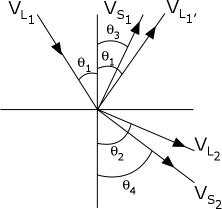SNELL'S LAW
Snell’s Law determines the path that sound energy takes when moving from one medium to another, for example from a borehole full of mud into a rock (as in well logging) or from one layer of rock to another (as in seismic reflection and refraction). The law states that:
19: sin (Incident Angle) / sin (Transmitted Angle)
= (Incident Velocity) / (Transmitted Velocity)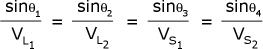Snell's Law applies to all electromagnetic waves as well as acoustic waves.

The critical angle is the angle of incidence that creates refraction of sound energy along the interface between two dissimilar media, for example along the wellbore face (as in well logging) or along the boundary between two rock layers (as in seismic refraction surveys). The equation is:
20: CritAngl = ArcSin (Incident Velocity) / (Transmitted Velocity)

For the sonic log:
21: CritAngl = ArcSin (Vmud / Vrock) = ArcSin (DTCrock / DTCmud)

Sonic logging tools consist of one or more sources of pulsed sound energy and a number of sound detectors. The sound travels from the source on the logging tool, through the mud in the borehole, to the rock. Here it is refracted at the critical angle, according to Snell’s Law, and travels in the rock parallel to the borehole.

The source creates a compressional wave through the mud, a portion of which undergoes mode conversion to create a shear wave as well as the compressional wave in the rock. The shear wave is slower than the compressional, and modern sonic log processing can segregate and record both.

Both compressional and shear waves refract back into the borehole, the shear converting to a compressional wave, to be detected by the receivers in the logging tool.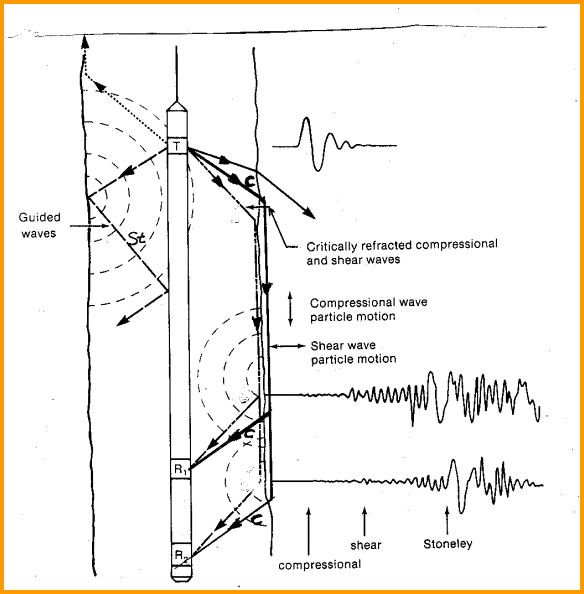Schematic diagram of a simple sonic log showing sound paths, following Snell's Law.Sound Velocity in Gases
The only sound wave passed through a gas is the compressional wave. Its velocity is:
22: Vgas = 68.4 * (Ks * P / DENS)^0.5

Where:
DENS = density (lb/cuft)
Ks = ratio of specific heats (unitless)
P = pressure (psi)
Vgas = velocity of sound (ft/sec)

Note that V is a Velocity here, while V is used as Volume elsewhere in this Handbook.Numerical Example:
Find the velocity of sound in a gas with same composition and under the same reservoir conditions as before, given the ratio of specific heats equal to 1.37.
Vgas = 68.4 * (1.37 * 1000 / 4.936) ^ 0.5 = 1140 ft / sec
DTCgas = 10 ^ 6 / 1,140 = 877 usec/ftSound Velocity in Liquids
Again, only the compressional wave is transmitted. Velocity of sound in water or oil can be derived from:
23: Vliq = ((144 * Gc) / (Cw * DENSliq)) ^ 0.5

Where:
Cw = compressibility of liquid (psi^-1)
DENSliq = density of liquid at downhole conditions  (lb/cuft)
Gc = acceleration of gravity = 32.7 ft/sec ^ 2
Vliq = velocity of sound in liquid (ft/sec)

Cw must usually be found from laboratory measurements.Numerical Example:
Find the sound velocity of oil and water from the previous example.
Voil = ((144 * 32.17) / (3.0*10^-6 * 71.70)) ^ 0.5 = 4,640 ft/sec
DTCoil = (10 ^ 6) / Voil = 206 usec/ft

Vwater = ((144 * 32.17) / (2.0*10^-6 * 78.80 )) ^ 0.5 = 5,450 ft/sec
DTCw = (10^6) / Vwater = 184 usec/ftSound Velovity in Solids
The velocity of longitudinal (compressional) waves in solids can be predicted from the following two equations.
24: Vp = 68.4 * (((K + 4/3 * N) / DENS) ^ 1/2)
OR: 24A: Vp = 68.4 * (((Y * (1 - N) / (DENS * (1 - 2 * N) * (1 - N)) ^ 1/2)

Where:
K = bulk modulus of elasticity (psi)
DENS = density (lb/cuft)
N = shear modulus or modulus or rigidity (psi)
Vp = compressional velocity (ft/sec)
Y = Young's modulus (psi)

The transverse (shear) wave velocity is defined by the following two equations:
25: Vs = 68.4 * ((N / DENS) ^ 1/2)
OR 25A: Vs = 68.4 * (((Y / DENS) / 2 * (1 + PR)) ^ 1/2)

Where:
DENS = density (lb/cuft)
N = shear modulus or modulus or rigidity (psi)
PR = Poisson's ratio (unitless)
Vs = shear wave velocity (ft/sec)

To translate these formulae into metric, convert density into gm/cc, velocity to Km/sec and the various moduli to megabars, and change the constant terms to 1.0. To convert moduli in megabars to psi, multiply by 6.89 * 10^-6. To convert megabars to Kilopascals, multiply by 10^4. Also note that 1 GigaPascal (GPa = 10^10 dynes/cm^2.Sound Velocity in Mixtures - WYLLIE TIME AVERAGE EQUATION
An equation similar to that for density can be generated for sound velocity of mixtures. It is a summation of travel time weighted by volume and not a summation of velocity components:
26: DTC = Sum (DTCi * Vi)

Where:
DTC = travel time of mixtures (usec/ft or usec/m)
DTCi = travel time of ith component (usec/ft or usec/m)
Vi = volume of ith component (fractional)

This is called the Wyllie time average equation and is true for many situations where the components are not very compressible, such as oil, water, sandstone, and shale. It does not work too well with gas under low pressure. This formula is an empirical relationship and is not rigorous, and is therefore, not a law of physics.

The expansion of this formula for log analysis parallels the density formula:
27: DTC = PHIe * (1 - Sw) * DTCh
+ PHIe * Sw * DTCw
+ Vsh * DTCsh
+ (1 - PHIe - Vsh) * DTCma

 Table 1:  KS8 – DTS / DTC Multiplier Coal 1.9 to 2.3 Shale 1.7 to 2.1 Limestone 1.8 to 1.9 Dolomite 1.7 to 1.8 Sandstone 1.6 to 1.7

28: KS8 = SUM (Vxxx * (DTS?DTCmultiplier))
29: DTSsyn = KS8 * DTCsyn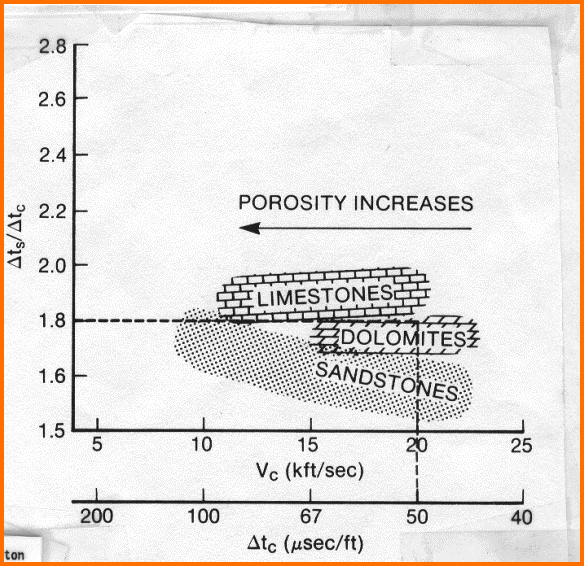Where:
DTC = compressional travel time of composite material (usec/ft or usec/m)
DTS = shear travel time of composite material (usec/ft or usec/m)
DTCh = travel time of hydrocarbon (usec/ft or usec/m)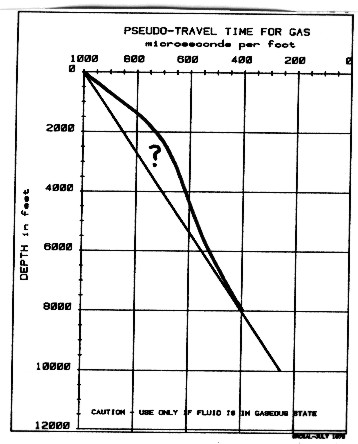DTCma = travel time of matrix rock (usec/ft or usec/m)
DTCsh = travel time of shale (usec/ft or usec/m)
DTCw = travel time of water (usec/ft or usec/m)
PHIe = porosity (fractional)
Sw = water saturation (fractional)
Vsh = shale volume (fractional)
Vxxx = volume of each mineral present, normalized so that
SUM(Vxxx) = 1.0
(DTS/DTCmultiplier) = Vp/Vs ratio for a particular mineral (Table 1)

This expansion works well as a predictive tool with oil or water in the porosity, as the travel time of sound in liquids is quite predictable.  Equation  21: Vgas = ((Ks * P) ^ 1/2) / DENS, where K is ratio of specific heats and P is pressure, looks like a good solution for finding DTCh for gas zones. However Ks is not usually available and some other solution is often needed. The Biot-Gassmann equations are often preferred, empirical values for DTCh are also used, as in the graph at the right..NUMERICAL EXAMPLES - Biot-Gassmann and Wyllie Equations
Combining equations 9 through 12, we get:
28: Kc = Kb + ((1 - Kb / Km) ^ 2) / (PHIe / Kf + (1 - PHI) / Km - Kb / (Km ^ 2))Hard Rock Example - Gassmann
Given:
Dolomitic limestone with 20% porosity at 10,000 feet
grain density = 2.78 gm/cc
acoustic velocity = 14,000 ft/sec at 100% water saturation = 71.4 usec/ft
bulk density = 2.44 gm/cc
formation water = 200,000 ppm total dissolved solids
reservoir temperature = 240 degrees F
reservoir pressure = 4700 psi

What percent change in velocity could be expected if 75% of the pore space were filled with methane gas?

0. Convert velocity to cm/sec.
Vp = 14000 * 0.3048 * 100 = 426720 cm/sec

1. Invert the velocity equation and solve for Kc (sometimes referred to as the space modulus M) for 100% water-bearing condition.
Kc = (Vp ^ 2) * DENS = 426750 * 426720 * 2.44 = 44.5*10^10 dynes/cm2 = 44.5 GPa

2. Determine Km from handbook data.
dolomite = 82 *10^10 dynes/cm2 (82 GPa)
limestone = 67 * 10^10 dynes/cm2 (67 GPa)

For this example, Km was estimated to be 74.5 * 10^10 dynes/cm2 (74.5 GPa) because the rock was a mixture of limestone and dolomite.

3. Estimate Kb.
Cb = 3.7*10^-6 psi-1 from handbook data
Kb = 1 / Cb = 1.86*10^10 dynes/cm2 (1.86 GPa)

4. Determine fluid properties at reservoir conditions.
Water compressibility (Cw) was estimated to be:
Cw = 2.26*10^-6 psi-1 from handbook data
Kw = 1 / Cw = 3.05 * 10^10 dynes/cm2 (3.05 GPa)

Water density = 1.085 gm/cc.
Gas density was estimated to be 0.157 gm/cc.

Gas compressibility (Cg) was computed from:
Cg = 161*10^-6 psi-1 from handbook data
Kg = 1 / Cg = 0.0428 * 10^10 dynes/cm2 (0.0428 GPa)

Gas-water combination properties.
DENSf = 0.25 * (1.085) + 0.75 * (0.157) = 0.389 gm/cc

Kf from the Wood equation.
1 / Kf = Sw / Kw + Sg / Kg
Kf = 0.0568*10^10 dynes/cm2 (0.0568 GPa)

5. Solve Gassmann equation for Kb at Sw = 100%.
Kb @ 100% water saturation = 44.5 * 10^10 - ((1 - 1.86*10^10 / 74.5 * 10^10) ^ 2) /
(0.2 / 3.05 * 10^10 + 0.8 / 74.5 * 10^10 - 1.86 / (74.5 * 10^10) ^ 2
= 32.9 * 10 ^ 10 dynes/cm2  (32.9 GPa)

6. Solve this equation for Kc with combination gas/water conditions.
Kc @ 75% gas saturation = 44.5 * 10^10 - ((1 - 1.86 * 10^10 / 74.5 * 10^10) ^ 2) /
(0.2 / 0.0568*10^10 / 74.5 * 10^10 - 1.86 / (74.5*10^10) ^ 2)
= 33.2*10^10 dynes/cm2  (33.2 GPa)

7. Solve density equation for gas/water combination.
DENS  @ 75% gas saturation = 0.2 * (0.389) + 0.8 * (2.78) = 2.30 gm/cc

8. Solve for Vp for Sg = 0.75.
Vp @ 75% gas saturation = (33.2 * 10^10) ^ 0.5 / 2.30 = 3.80 * 10^5 cm/sec = 12,500 ft/sec

Utilizing this methodology and the stated assumptions, there would be an approximate 10% change in velocity (14,000 - 12,500 / 14,000) from a 100% water saturated zone to one that has identical rock properties but 75% gas saturation in the pore space. As a matter of interest, if the rock frame compressibility were assumed to be 5.0 * 10^-7 psi-1, the computed gas/water-bearing velocity would be 13,100 ft/sec. This is only 7% slower than the observed water-bearing velocity and helps demonstrate the sensitivity of the calculation to the assumption of rock frame modulus.Soft Rock Example - Gassmann
Given:
Tertiary sandstone with 32% porosity.
grain density = 2.65 gm/cc at 7,000 ft
acoustic velocity = 8,130 ft/sec = 123 usec/ft
bulk density = 2.17 gm/cc at 100% water saturation
formation water = 225,000 ppm TDS
reservoir temperature = 184 degrees F
reservoir pressure = 7,300 psi
estimated net effective stress < 1,000 psi

What percentage change in velocity could be expected if 75% of the pore space were filled with methane gas (gas gravity 0.8)?
Kc = 13.3 * 10^10 dynes/cm2  (13.3 GPa)
Km taken as 37.9 * 10^10 dynes/cm2  (37.9 GPa)
Estimated Cb = 3.5 * 10^-6 psi^-1 from experience.

Compressibility values on poorly consolidated formations are difficult to obtain. It is believed that many operators are attempting to measure and catalogue such data. However, most of these data are being held confidential.
Kb = 1 / Cb = 1.97 * 10^10 dynes/cm2  (1.97 GPa)
Kw = 3.71 * 10^10 dynes/cm2  (3.71 GPa)
Kg = 0.186 * 10^10 dynes/cm2  (0.186 GPa)
Kf = 0.244 * 10^10 dynes/cm2
DENSg = 0.32 gm/cc
DENSw = 1.15 gm/cc
DENSf = 0.528 gm/cc
Kb @ 100% water saturation = 4.59 * 10^10 dynes/cm2  (4.59 GPa)
Kc @ 75% gas saturation = 5.27 * 10^10 dynes/cm2  (5.27 GPa)
DENS @ 75% gas saturation = 1.97 gm/cc
Vp @ 75% gas saturation = 5,370 ft/sec

The velocity change computed with these assumptions is approximately 34%. However, the computed velocity of 5,370 feet per second, or 186 microseconds per foot, compares reasonably well to observed sonic log values in gas zones having rock and reservoir properties similar to the assumptions made in this calculation.

As a matter of interest, if the bulk compressibility of the rock frame is assumed to be 3.0 * 10^-5 psi-1, or an increase of nearly an order of magnitude, the computed Gasmann velocity for 75% gas is 5,000 feet per second. This is an additional 4.5% decrease in velocity from the water-bearing case.Hard Rock Example - Wyllie
Same rock properties as Case 1:
porosity (PHIe) = 0.20
shale content (Vsh) = 0.00
water saturation (Sw) = 0.25
shale travel time (DTCsh) = 70 usec/ft
matrix travel time (DTCma) = 44 usec/ft
water travel time (DTCw) = 189 usec/ft
gas pseudo-travel time (DTCh) = 250 usec/ft

Solving the equation:
DTC = (1 - 0.20 - 0.00) * 44 + 0.00 * 70 + 0.20 * 0.25 * 189 + 0.20 * 0.75 * 250
= 82.15 usec/ft
Vp = 10^6 / DELT = 12,170  ft/sec
(compared to 12,500 ft/sec for Gasmann equation)Soft Rock Example - Wyllie
Same rock properties as Case 2:
porosity (PHIe) = 0.32
water saturation (Sw) = 0.25
shale volume (Vsh) = 0.10
shale travel time (DTCsh) = 90 usec/ft
matrix travel time (DTCma) = 55.5 usec/ft
water travel time (DTCw) = 189 usec/ft
gas pseudo-travel time {DTCh) = 550 usec/ft

Solving the equation:
DTC = (1 - 0.32 - 0.10) * 55.5 + 0.10 * 90 + 0.32 * 0.25 * 189 + 0.32 * 0.75 * 550
= 188.3 usec/ft
Vp = 10^6 / 188.3 = 5,310 ft/sec
(compared to 5,370 ft/sec for Gasmann equation)

Note that the result depends largely on the choice of gas pseudo-travel time, and this is subject to some error in judgment.

Page Views ---- Since 01 Jan 2015
Copyright 2023 by Accessible Petrophysics Ltd.
CPH Logo, "CPH", "CPH Gold Member", "CPH Platinum Member", "Crain's Rules", "Meta/Log", "Computer-Ready-Math", "Petro/Fusion Scripts" are Trademarks of the Author